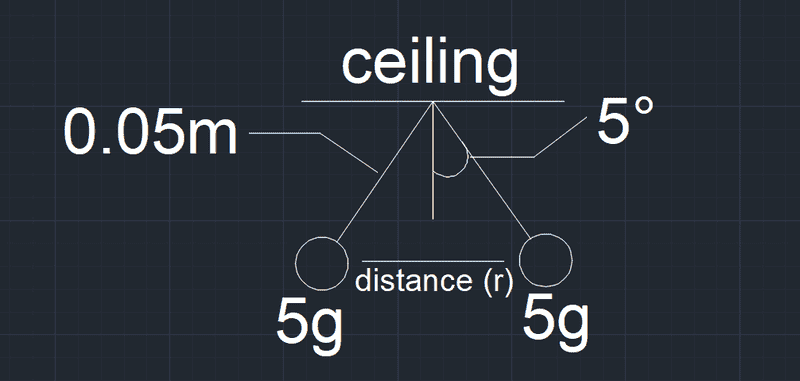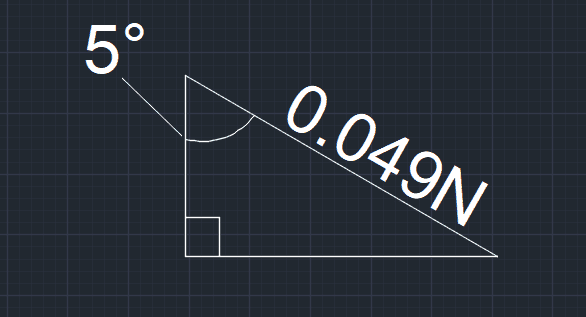# Coulomb's Law and Two Balls

• Civil_Disobedient
In summary: Where did you get that?In summary, the conversation discusses a problem involving two small balls with a mass of 5.0 g each, attached to silk threads that are tied to the same point on the ceiling. When given the same charge Q, the balls hang at an angle of 5.0° to the vertical. The conversation includes equations such as Coulomb's Law and F = mg to determine the magnitude of Q. The correct solution is found to be 9.17 * 10^-7C. However, there is a discussion about torque and using it to solve the problem, rather than determining the tension in the string.

## Homework Statement

Two small balls, each of mass 5.0 g, are attached to silk threads 50 cm long, which are in turn tied to the same point on the ceiling, as shown below. When the balls are given the same charge Q, the threads hang at 5.0° to the vertical, as shown below. What is the magnitude of Q? What are the signs of the two charges?

## Homework Equations

Coulomb's Law: F = k*q^2 / r^2

## The Attempt at a Solution

Here's the drawing of the problem:So far I've found distance r by dividing each half into 2 triangles and using trig:
0.05 * sin5 = r/2
r = 0.0087m

To find Q, I rearranged Coulomb's Law like so:
F = k*q^2 / r^2
F*r^2 = k*q^2
q = sqrt(F*r^2 / k)

To find force, I used F = mg
F = 5g * 10 = 50N per ball, 100N total for two balls

From there, I plugged in what I got
q = sqrt(F*r^2 / k
q = sqrt(100*0.0087^2 / 9*10^9)
q = 9.17 * 10^-7C

So I ended up with 9.17 * 10^-7C, which I feel is wrong (I suspect I may have calculated force wrong) but I don't have an answer or solution key and sadly can't check mines or any other solutions. Any help is appreciated!

#### Attachments

Last edited:
50 cm is not 0.05 m
5 g is not 50 N, and you don't add the two balls together.
Is F equal to mg? Consider that they are acting in orthogonal directions.
Take one ball, and consider the torque on it.

•Civil_Disobedient and berkeman
mjc123 said:
50 cm is not 0.05 m
5 g is not 50 N, and you don't add the two balls together.
Is F equal to mg? Consider that they are acting in orthogonal directions.
Take one ball, and consider the torque on it.

I figured out some of the incorrect unit conversions I made. 50 cm = 0.5 m, so r = 0.087.
5g = 0.005 kg --> 0.049 N
But I don't quite get the part about torque. I've tried torque = rFsinθ.
For q = sqrt(F*r^2 / k), shouldn't I use force of tension in the diagonal direction instead of torque?

Civil_Disobedient said:
I've tried torque = rFsinθ.
I assume you are taking that from a generic equation where those three variables are defined suitably, but they are not the same as the three variables of the same names in the present problem.
@mjc123 means consider the torque balance on a ball+string system about the point where the string is attached to the ceiling. What forces have a torque about that point?

•Civil_Disobedient
Okay, so that force would be the horizontal force of tension. This creates a right triangle with angle 5 degrees and a hypotenuse of 0.049N, which is the tension. Using trig, 0.049N * sin(5) = 0.0043N (horizontal tension). From there, I ended up with 6.01 * 10^-8C for charge.I used the solutions to help a bit on this last part with tension and horizontal tension. My last question is how did you find the force of tension to be 0.049N? Was it just F = mg = 0.05 kg * 9.8 m/s^2?

#### Attachments

Civil_Disobedient said:
so that force would be the horizontal force of tension.
No, the tension acts through the point of contact of the string with the ceiling, so has no torque about that point. Which forces on the ball do have a torque about it?
Civil_Disobedient said:
hypotenuse of 0.049N, which is the tension
Nobody said the tension was 0.049N. The point of mjc's method is to avoid having to determine the tension. If you are not comfortable with that method, just write out the usual two equations for horizontal and vertical balances of forces, using an unknown for the tension.

•Civil_Disobedient
Civil_Disobedient said:
My last question is how did you find the force of tension to be 0.049N? Was it just F = mg = 0.05 kg * 9.8 m/s^2?

Nope, that's nothing at all. Nothing is 0.05 kg.